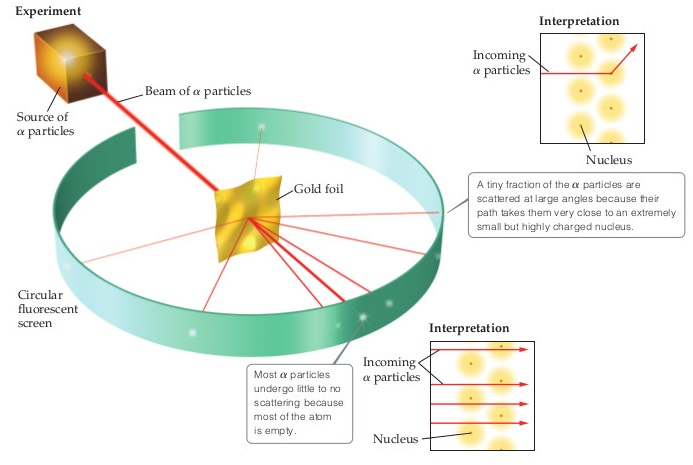# Problem: You may want to reference (Pages 46 - 49) Section 2.2 while completing this problem.What fraction of the α particles in Rutherford's gold foil experiment are scattered at large angles? Assume the gold foil is two layers thick, as shown in the figure, and that the approximate diameters of a gold atom and its nucleus are 2.2 Å and 1.0 × 10− 4 Å, respectively. Hint: Calculate the cross-sectional area occupied by the nucleus as a fraction of that occupied by the atom. Assume that the gold nuclei in each layer are offset from each other.

###### FREE Expert Solution###### Problem Details

You may want to reference (Pages 46 - 49) Section 2.2 while completing this problem.What fraction of the α particles in Rutherford's gold foil experiment are scattered at large angles? Assume the gold foil is two layers thick, as shown in the figure, and that the approximate diameters of a gold atom and its nucleus are 2.2 Å and 1.0 × 10− 4 Å, respectively. Hint: Calculate the cross-sectional area occupied by the nucleus as a fraction of that occupied by the atom. Assume that the gold nuclei in each layer are offset from each other.